# 【MFC】多线程计算圆周率π的值

1、新建一个基于对话框的mfc应用程序。2、新建一个对话框。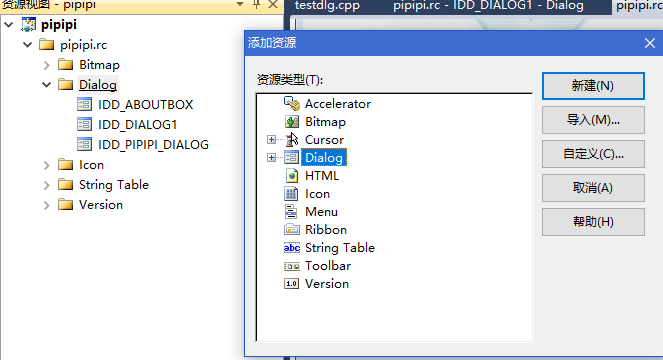3、然后就是从右侧工具栏拉进来必要的组件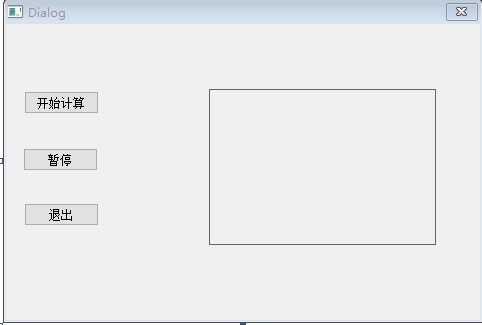4、编码。

void CpipipiDlg::OnBnClickedButton1()
{
testdlg *test = new testdlg;
test ->Create(IDD_DIALOG1,this);
test ->ShowWindow(SW_SHOW);

// TODO: 在此添加控件通知处理程序代码
}

void testdlg::OnBnClickedButton2()
{
//AfxMessageBox(_T("开始了"));

DWORD   dw1;

for(;b-c;) f[b++]=a/5;
for(;d=0,g=c*2;c-=14,temp[i++]=e+d/a,e=d%a)
for(b=c;d+=f[b]*a,f[b]=d%--g,d/=g--,--b;d*=b);
SetTimer(1,300,NULL);
i=0;
// TODO: 在此添加控件通知处理程序代码
}

static DWORD WINAPI ThreadB1(LPVOID lpParam)
{
lock=1;
return 1;
}

int i=0;
CString ss,cc;
long a=10000,b,c=2800,d,e,f,g;
int temp;

void testdlg::OnBnClickedButton3()
{

// TODO: 在此添加控件通知处理程序代码
DWORD   dw2;

}

static DWORD WINAPI ThreadB2(LPVOID lpParam)
{
lock=0;
return 1;
}

void testdlg::OnTimer(UINT_PTR nIDEvent)
{
// TODO: 在此添加消息处理程序代码和/或调用默认值
if(n==0)
ss+="3.141",i++;
else
ss+=cc;
n++;
cc.Format("%04d",temp[i++])  ;
if(n%5==0)
ss+="\n";

SetDlgItemText(   IDC_STATIC1,   ss);

if(lock==0)
KillTimer(1);
CDialogEx::OnTimer(nIDEvent);
}
n初始值为0，第一次进入的时候，我们直接定位3.141，否则原本的计算方法只是3141，没有小数点。除此之外的情况下，每次ss后面补充当前4位cc的字符串，而cc每次从保存结果的temp[]数组中获取4位当前值。

5、点缀。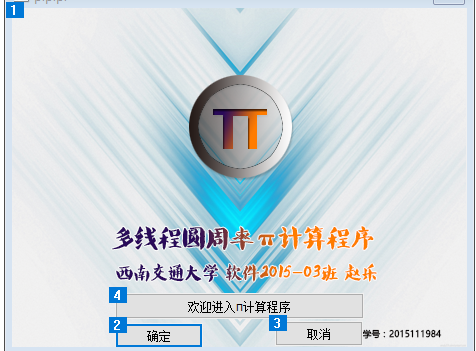SetBackgroundImage(IDB_BITMAP2);设置背景图的，感觉这里拉大小和位置太麻烦了，原图来自千库网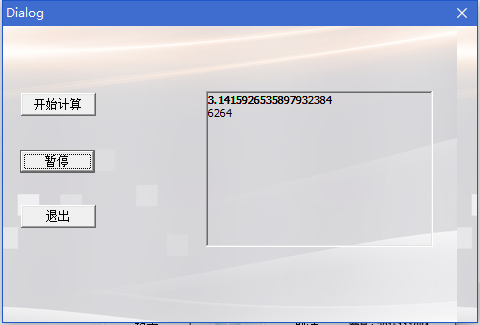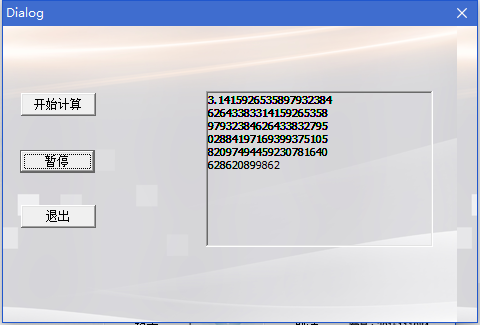6、最后讲讲更换图 标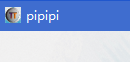OK！